GeeksforGeeks App
Open AppBrowser
Continue

# How HashTable Works Internally in Java?

Hashtable is a kind of Hash map but is synchronized. Hash map is non–synchronized, permits one null key & multiple null values, not-thread safe i.e. cannot share between many threads without proper synchronization,  the key/values pairs are stored in Hashtable. The Hash table does not allow null value/key. When using this you specify an object that is used as a key & the value that you want to associate with the key. It is an array of an index. Each index is known as a bucket/slot. It restrains values based on keys & the place of bucket recognized by calling the Hash code () method. The Hash table of Java holds a class that has unique elements.

Working of Hashtable

Hash table intrinsically contains a slot/bucket in which the storage of key and value pair. It uses the key’s hash code to discover which bucket the key/value of a set should map. To find an item in a list you do the first approach i.e. linear search this involves checking each item, it will take more time. Suppose you have retrieved a value and its index number of an array, then you will look up a value very quickly. In fact, a time will be taken less if you know the index number is independent of the size & position of the array. But how do you know which element of the array contains the value you are looking for. The answer is by calculating the value itself the index number is somewhat related to data.

Let’s proliferate the array, take a name and find its ASCII code, for example, we will take a name say Mia find ASCII code for each letter & add the ASCII code and divide the combined ASCII number with the size of an element in this it is 5, we will take a reminder of the element that is 4 and there we will put the name Mia i.e. in 4th Slot/ Bucket.

Example:

Name = Mia

M  77

i 105

a  97

Sum = 279

Modulus = 279 % 5 = 4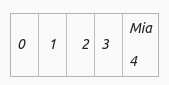And now we can add more names to the Bucket as shown below:

Mia ⇾ M (77) + i(105) + a(97) = 279 % 5 = 4

Tim ⇾ T (84) + i(105) + m(77) = 298 % 5 = 3

Leo ⇾ L(76) + e(101) + n(110) = 287 % 5 = 2

Som ⇾ S(83) + o(111) + m(77) = 271 % 5 = 1

Beb ⇾ B(66) + e(101) + b(98) = 265 % 5 0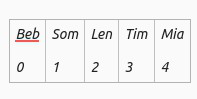Index number = sum of ASCII codes % Size of array

Let’s retrieve an item say Ada we perform the same calculation,

Find ASCII for Ada = (65+100+97) = 262

262 % 11 = 9

MyData = Array (9)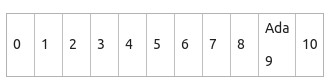This is a fast array lookup. Rather than storing individual items or data hash tables are used to store the key-value pairs. For example, Ada would be the key for which want to calculate the Index and DOB of the corresponding value, for this reason, a hash table of key-value pair is sometimes referred to as a hash map in fact if an object-oriented approach is taken each person in an instance of a class and the key would be of many properties, by populating an array with objects you will effectively store much-related data you like for each bucket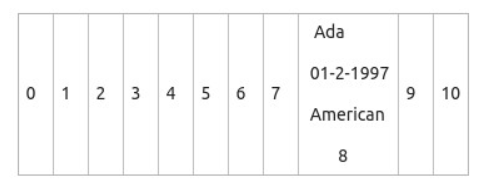A hashing algorithm also is known as a Hash function. The hash function is a function that generates a table when given a key. This is a function to obtain a bucket location from the Key’s hash code. It every time returns a digit for an object. Calculation applied to a key to transforming it into an address.

For numeric keys, divide the key by the number of available addresses, n, and take the remainder

For alphanumeric keys, divide the sum of ASCII codes in a key by the number of available addresses, and take the remainder.

• Folding method divides key into equal parts then adds the parts together
• Phone No. 018767242947 becomes 01+87+67+24+29+47= 255
• Depending on the size of a table, may then divide by some constant and take the remainder.
• There are lots of Hash functions some appropriate to another depending on nature.

Collisions in a Hashtable

So far you have seen, there are a lot of a hash table with data that is very conveniently causing any problem say that is unrealistic sometimes if you apply a hash function in two different cases it generates the same index number for both but both items can’t go in the same place this is called Collision. Two identical objects every time have the same digits while two unequal objects might not always have dissimilar digits. Putting an object to the hash table, there is a possibility that dissimilar objects might have an equal hash code called Collision. For rectifying this array of lists is used by a hash table. The set mapped to array index/single bucket and is kept in an index & index reference is stored in array index.

Let’s load up the array again this time with a different set of data. As shown below if Mia is occupying position 4 and Leo to position 2 and if the other names too have the same entry at position 2 then automatically the next bucket will be filled with that entry for avoiding collision as shown below.

Find Rae 280 Mod 11 = 5

myData = Array(5)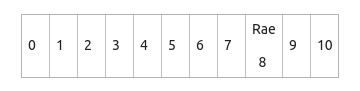As the reminder for an ASCII code of Rae is 5 but is put in slot 8 because due to collision the 2 items figure out to be in the same slot but for avoiding this the Rae is shifted to other slot and as the other slot is again occupied so it is shifted to the 8th position.

Resolving a collision by putting the item somewhere else then its calculated address is called OPEN ADDRESSING because every location is open to an item. This can use a variety of techniques to decide where to place an item this particular addressing technique is called LINEAR PROBING if the calculated address is occupied then the linear search is used to find the next available bucket if the linear probing comes to an end and still cannot find a position it might cycle around the beginning of array & continue searching from there.  The more items there are in a hash table the more chances of a collision. One way to deal with this is making the hash table bigger for the total amount of data you are expecting perhaps such that happen 70% of the table is occupied the ratio between the no of items stored and the size of an array is known as Load Factor

Load Factor = Total number of items stored / Size of the array

Another way to do with collisions is known as chains sometimes referred to as CLOSED ADDRESSING. In this, the one slot can form a chain by adding the various item into one bucket.

The objective of a Hash Function

• Minimize collisions
• Uniform distribution of hash values
• Easy to calculate
• Resolve any collisions

Methods like hashCode () & equal () to find exact element match

equal(): Object class define equal() as a given language of Java. In which objects indicate whether few objects pass as an argument that is “equal to” the present instance. The different or two objects can be similar only if they are put on an equal memory address.

Syntax:

```public boolean equals  (Object obj)

// This method checks if some other Object
// passed to it as an argument is equal to
// the Object on which it is invoked.```

• Reflexive: For a single object say a, a.equal(a) return true.
• Symmetric: For two different objects, a.equal(y) return true if and only if y.equal(a) returns true.
• Transitive: For multiple objects a, b, c if a.equal(b) returns true & b.equals(c) returns true then a.equals(c) should return true.
• If the object properties are modified means used in equal() method implementation this will result in different and multiple of a.equal(b) will return equal outcome.
• Object class equal() will return true only when two references will mark similar objects.

Note: For any non-null reference value a, a.equals(null) should return false.

hashCode(): In this object gives back an integer depiction of an object’s memory address. By using this method a random integer will get returned that is unique for a particular instance. This will return integer hash code data or value of that object.

Syntax:

```public int hashCode()

// This method returns the hash code value
// for the object on which this method is invoked.```

Example:

## Java

 `import` `java.util.*;` `public` `class` `HashCodeExample {``    ``public` `static` `void` `main(String[] args)``    ``{``        ``// develop integer object``        ``Integer i = ``new` `Integer(``"144"``);` `        ``// Returned hash code value``        ``int` `hashValue = i.hashCode();` `        ``System.out.println(``"Value of Hashcode : "``                           ``+ hashValue);``    ``}``}`

Output

`144`

Steps

• A base class has attributes for objects for user define.
• By using @override the override hashCode() function can be predefined.
• In a similar way, the override equal() function can be predefined.
• Declare an object of the base class and use hash code and equals function so that objects would be equal in the main function.

Contract of hash code() and equal()

• During the execution of the application, if hashCode() is invoked more than once on the same Object then it must consistently return the same Integer value, provided no information used in equals(Object) comparison on the Object is modified. It is not necessary that this Integer value remain the same from one execution of the application to another execution of the same application.
• If two objects are equal, according to the equals(Object) method, then hashCode() method must produce the same Integer on each of the two Objects.
• If two Objects are unequal, according to the equals(Object) method, It is not necessary the Integer value produced by hashCode() method on each of the two Objects will be distinct. It can be the same but producing the distinct Integer on each of the two Objects is better for improving the performance of hashing based Collections like HashMap, HashTable, etc.

Note: Equal objects must produce the same hash code as long as they are equal, however unequal objects need not produce distinct hash codes.

### Uses

It is a data structure that executes an associative array transcendent data type, a structure that can map keys to values. Here we use the Hash Algorithm to determine a list called hash code into an array of Slots from which desired values can be obtained. Hash table is used to implement an in-memory table, in a programming language like python, PHP, etc., in error checking. An associative array, database indexing, caches, sets, objects representation, unique data representation, Transposition table, etc.

Example 1:

## Java

 `import` `java.util.*;` `public` `class` `HashtableExample {``    ``public` `static` `void` `main(String[] args)``    ``{``        ``// Create Hashtable``        ``Hashtable hashtable``            ``= ``new` `Hashtable<>();` `        ``// Add mappings to hashtable``        ``hashtable.put(``1``, ``"X"``);``        ``hashtable.put(``2``, ``"Y"``);``        ``hashtable.put(``3``, ``"Z"``);``        ``System.out.println(hashtable);` `        ``// Get a mapping by key``        ``String value = hashtable.get(``1``);` `        ``System.out.println(value);` `        ``//  Remove a mapping``        ``hashtable.remove(``3``);` `        ``// Iterate overmapping``        ``Iterator itr``            ``= hashtable.keySet().iterator();` `        ``while``(itr.hasNext())``        ``{``            ``Integer key = itr.next();``            ``String mapped_value = hashtable.get(key);``            ``System.out.println(``                ``"key: "` `+ key + ``" value : "` `+ mapped_value);``        ``}``    ``}``}`

Output

```{3=Z, 2=Y, 1=X}
X
key: 2 value : Y
key: 1 value : X```

Example 2:

## Java

 `import` `java.util.Hashtable;``import` `java.util.Enumeration;``public` `class` `HashtableExample {` `    ``public` `static` `void` `main(String[] args)``    ``{` `        ``Enumeration names;``        ``String key;` `        ``// Developing a Hashtable``        ``Hashtable hashtable``            ``= ``new` `Hashtable();` `        ``// Attaching Key and Value sets to Hashtable``        ``hashtable.put(``"Key1"``, ``"Madhu"``);``        ``hashtable.put(``"Key2"``, ``"Girja"``);``        ``hashtable.put(``"Key3"``, ``"Durgesh"``);``        ``hashtable.put(``"Key4"``, ``"Richa"``);``        ``hashtable.put(``"Key5"``, ``"Manisha"``);` `        ``names = hashtable.keys();``        ``while` `(names.hasMoreElements()) {``            ``key = (String)names.nextElement();``            ``System.out.println(``"Key: "` `+ key + ``" & Value: "``                               ``+ hashtable.get(key));``        ``}``    ``}``}`

Output

```Key: Key4 & Value: Richa
Key: Key3 & Value: Durgesh
Key: Key2 & Value: Girja
Key: Key5 & Value: Manisha```

Conclusion

• Used in index large amounts of data
• Address of each key calculated using the key itself
• Collisions resolved with open or closed addressing
• Hashing is widely used in database indexing, compilers, caching, password authentication, and more
• Insertion, deletion, and retrieval occur in constant time.

My Personal Notes arrow_drop_up